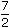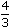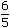# Aptitude - Partnership - Discussion

### Discussion :: Partnership - General Questions (Q.No.3)

3.

A, B and C enter into a partnership in the ratio::. After 4 months, A increases his share 50%. If the total profit at the end of one year be Rs. 21,600, then B's share in the profit is:

 [A]. Rs. 2100 [B]. Rs. 2400 [C]. Rs. 3600 [D]. Rs. 4000

Explanation:

 Ratio of initial investments =7 : 4 : 6= 105 : 40 : 36. 2 3 5

Let the initial investments be 105x, 40x and 36x.A : B : C =105x x 4 + 150 x 105x x 8: (40x x 12) : (36x x 12) 100

= 1680x : 480x : 432x = 35 : 10 : 9.

 Hence, B's share = Rs.21600 x 10= Rs. 4000. 54

 Anurag said: (Oct 19, 2010) As per question A increase his share 50%, it means A's contribution Contribution 4 moth 105*4 = 420 A's contribution for 8 month will be 105*8 = 840 50% increase of 840 is 1260 For 12 month = 1260 + 420 = 1680.

 Priya said: (Nov 30, 2010) @Anurag. Thank for good explanation. Really helpful.

 Md Rizwan said: (May 5, 2011) 7 : 4 : 6 = 105 : 40 : 36. 2 3 5 Plz Explain

 Usha said: (Jun 4, 2011) Please explain that 105:40:36 how you are taken this.

 Pradeep Induru said: (Jun 17, 2011) How could you get that 105:40:36?

 Ally said: (Jun 17, 2011) Please explain from where did you get. 105: 40: 36.

 Senthil said: (Jun 22, 2011) 105:40:36 how ? Ans: Take lcm for 2,3,5 Hhich is in denomonator LCM = 30 Then 30*(7/2)=105; like this we can get for remaining.

 Ravindra said: (Jul 28, 2011) 7 : 4 : 6 = 105 : 40 : 36. 2 3 5 Plz Explain me

 Manoj Rocky said: (Aug 4, 2011) 7/2 :4/3 :6/5 Take L.C.M FOR 2,3,5. ANS:30 A: 7/2 * 15/15 =105/30 B: 4/3 * 10/10 =40/30 C: 6/5 * 6/6 =36/30 Hence it is 105:40:36 I hope you got the answer.

 Dhivya said: (Aug 12, 2011) A : B : C = 105x x 4 + 150 x 105x x 8: (40x x 12) : (36x x 12) = 1680x : 480x : 432x = 35 : 10 : 9. Anyone explain this step i didn't understand.

 Saroj said: (Aug 24, 2011) Thank you manoj.

 Kalam said: (Sep 3, 2011) Please explain how we got 105:40:36?

 Lechisagadisa said: (Sep 17, 2011) Find the minimum number that will be divide 2,3 and 5 simultaneously. That is known as LEAST COMMON DENOMINATOR.It will be 30. Then 7*15/30,4*10/30,6*6/30. BUT 15,10,6 are by dividing(the given denominator 2,3,5 with LCM=30). Then we can avoid the common denominator 30,and Finally we can get 105=15*7, 40= 4*10, and 36= 6*6. Thank you Rocky.

 Divya said: (Sep 29, 2011) First divide 1680:480:432 by 48,we wil get 35:10:9 then add al of them we wil get 54 then divide [21600*10 /54]=ans

 Mukund said: (Oct 3, 2011) I can't find sufficent explanation of this ans please exsplain again Let the initial investments be 105x, 40x and 36x. A : B : C = 105x x 4 + 150 x 105x x 8 : (40x x 12) : (36x x 12) 100 = 1680x : 480x : 432x = 35 : 10 : 9. Hence, B's share = Rs. 21600 x 10 = Rs. 4000. q 54

 Rajinikanth.H.C said: (Dec 3, 2011) A=(105*4months)+((105*8months)+50%(105*8months)) =420+(420+840) =1680 B=40*12months =480 C=36*12months =432 now u devide the above values by 48(all 3 values r devided without any fraction value)then u will get 35:10:9.... then add all 3 shares 35+10+9=54 for B share=21600*(B share/total share) =21600*(10/54) =4000rs

 Ranjeet said: (Jan 4, 2012) There is something wrong in answer if A increase his share it will also change the ratio of share others mean it would not be 40x:36x for B and C. Is anybody here clear my doubt?

 Alekhya said: (Jan 27, 2012) Here total profit is getting after 1 year i.e,, 12 months so 'a' increases profit after 4 months rest of the months is 8 answer increses to 50% so that we got 105x x 4+ 105x x 8+ 150/100 and rest of them for B and C ie for 12 months.

 Prerna said: (Mar 10, 2012) Hey clear my doubt why a is multiply with 8 and why why we take 50% of 8 yet here is only ask about the 50% of 4 month. Then why on 8 ?

 Yashal said: (Mar 13, 2012) Please explain why we multiply a's share with 150/100 ? 105x x 4 + (150/100) x 105x x 8

 Lavanya said: (Mar 15, 2012) Can anyone explain why we multiply a's share with 150/100? 105x*4+ (150/100) *105x*8.

 J.K Tamta said: (Mar 23, 2012) 7/2 of A's share for 4 month , 7/2*4= 14. and the remaining (14+7/4+7/2)*8= 56.EXPlAIN his share 7/2 kept for 4 month in business that is 14 . and 50% he increses after 4 month that is 7/4 now his share = 7/2+7/4*8 =42 , now 14+42=56 same as for B that is 4/3*12=16 and for C 6/5*12=14.4 now ratio is 56:16:14.4 = 86.4 B's share 16/86.4*21600= 4000 ANSWER

 Anu said: (Apr 11, 2012) Why we want to divide this ratio 160x:480x:432x by 48 only not by any number.

 Shashi said: (Sep 9, 2012) Can anyone explain why we multiply a's share with 150/100? 105x*4+ (150/100) *105x*8.

 Vikas said: (Sep 10, 2012) @ lavanya. A increased his stake by 50% so it will be 100+50=150% i.e. 150/100.

 Manish Singh said: (Feb 28, 2013) @Prerna. There we have to take the year as a 1 year means 12 month so already in question after 4 month means renaming month we have to take (12-4=8) for that 50% that we have to take for remaining month and we have to exclude 4 month because after 4 month only he invest.

 Sravani said: (Jul 22, 2013) Hi friends its very easy. Has given in question first two steps you are understood. 3rd step is the total profit at the end of 1 year 21600. So. (105*4+150/100*8) (40*12) (36*12). After 4 months means 105*4. 15% increase means 150/100 we have to take, 1680 480 432 35 10 9 total 54. 54......21600 10.......? 21600*10/54 = 4000.

 Sangeethamonica said: (Oct 23, 2013) Why should we divide by 48?. Have doubt can you explain.

 Joseph Sabar said: (Nov 24, 2013) How can I remember to the short form of the Partnership of Mathematics solved it my problem?

 Dushyant Verma said: (Feb 7, 2014) A's share of 4 month = 7/2 * 4 = 14. A's share of 8 month = (50% of 7/2)*8 = 21/4*8 = 42. A's share total 12 months = 14 +42 = 56. B's share total 12 months = 4/3 *12 = 16. C's share total 12 months = 6/5 *12 = 14.4. Now, B's profit = 21600*(16/86.4) = 4000.

 Nani said: (Mar 10, 2014) @Shashi. 105x*4+ (150/100) *105x*8. Step 1: first 4 months. Step 2: + 50% increase(150/100)*8months (remaining 8months adding) total one year. Multiply: 105x*4 = 420x. (150/100)*105x*8 = 1.5* 840x= 1260x. 420x+120x = 1680x.

 Abhishek said: (Nov 19, 2014) A : B : C = 105x x 4 + 150/100 x 105x x 8 : (40x x 12) : (36x x 12) From where this 150/100 is coming?

 Randeep Rajput said: (Dec 17, 2014) Its very easy like for A for 4 month: = 4*105 = 420. For 8 month. 8*105 = 840. Now 50% increase. 150/100*840 = 1260. Total A become 1260+420 = 1680.

 Fanciulladelwest said: (Jan 29, 2015) Can you tell me for what reason we use "x" when dealing with this problem? What does it state for? And how do we get rid of it in the last operation?

 Ankush said: (Jun 29, 2015) I think the answer is wrong. If A increases his share, B and C share would also get affected.

 Priya14 said: (Jul 9, 2015) How 150/100 came? Can anyone explain me briefly?

 Dinesh Reddy said: (Jul 30, 2015) They said that the share of A increases by 50 % so, the new total share of A be (100+50) =150, so 150%=150/100. And the 8 be the months as after 4 months so 4+4=8 months.

 Mohit God said: (Sep 1, 2015) Is there not any shortcut of such type of questions?

 Sunny said: (Oct 16, 2015) A : B : C = 105x x 4 + 150 x 105x x 8 : (40x x 12) : (36x x 12). How it will comes?

 Shubham said: (Oct 19, 2015) Short cut not available brother.

 Muhammed Afees said: (Oct 26, 2015) Total profit calculated for 1 year. So 1 year = 12 month. Partnership ratio 7/2:4/3:6/5, we need to expand this ratio. i.e, 7/2:4/3:6/5. In this we need to take 30 = 2*3*5 common factor based on which we need expand. A = 7/2 = 15*7/15*2 = 105/30. B = 4/3 = 10*4/10*3 = 40/30. C = 6/5 = 6*6/5*6 = 36/30. Hence expand ratio = 105:40:36. (105*4) + (105*150/100) *8) : (40*12) : (36*12).

 Manisha said: (Nov 17, 2015) How you got 105:40:36?

 Bhuvana said: (Nov 27, 2015) Can anyone explain me why we calculate the share for 1 year? Here it gave for only 4 months. Then A's share will be increase by 50%. I didn't understand.

 Nagu said: (Jan 5, 2016) As per question A increase his share 50%, it means A's contribution. 1 year = 12 month. 4 month 105*4 = 420. Remaining 8 month is 105*8 = 840.

 Likita said: (Jun 7, 2016) Why 840 is calculated for 50% of A's share and why not 420?

 Udhaya said: (Jun 21, 2016) First find the ratio. Which is 105:40:36? They have given A's share increases after 4 months which means for first 4 months it remains same and the next 8 months (as there are 12 months in a year) it increases by 50%, so we need to calculate first for 4 months separately and the for next 8 months after increasing by 50%. A's contribution for 4 months = 105 * 4 = 420. A's contribution for remaining 8 months = 105 * 8 = 840. As A's share increases in this 8 months, we need to calculate it, 840 + (840/2) = 840 + 420 = 1260. 50% increase means 840/2. Finally for a year=420+1260=1680. 50% increase of 840. So, 840 + (*820/2) = 820 + 420 = 1260.

 Ravi Chendra V said: (Jul 12, 2016) I had a confusion, A: 7/2 * 15/15 = 105/30 B: 4/3 * 10/10 = 40/30 C: 6/5 * 6/6 = 36/30 Hence it is 105:40:36 Here, How we get 15/15 and 10/10 and 6/6 can anyone explain this?

 Siva said: (Sep 8, 2016) I also have the same Confusion @Ravi Chendra.

 Gobi said: (Oct 6, 2016) Yeah, I also have the same doubt @Ravi Chendra.

 Angel said: (Jan 2, 2017) I am not getting this solution, please explain me.

 Jayasakthi said: (Jan 11, 2017) Can you please explain why we are changing the ratio into the whole number like 150?

 Nikhita said: (Jan 16, 2017) A:B:C = 7/2:4/3:6/5. Take lcm of 2,3,5 which will be 30 = 7 * 15 : 4 * 10 : 6 * 6, =105x : 40x : 36. A share for four months =105 * 4 = 420x. A share after 8 months= 105 * 8 = 840x. With 50 increase of total share. If share is 100 then A share increases of 50 % = 100 + 50 = 150/100. Therefore, A share for 8 months wth increased share = 840 * 150/100 = 1260. A share for 12 months = 1260 + 420 = 1680x. B share for 12 months = 40x * 12 = 480x. C share for 12 months = 36x * 12 = 144x. Ratio of A : B : C = 1680x : 480x : 144x 35x : 10x : 9x. Total profit = 21600. B share = 21600 * 10/54. = 4000RS.

 Alan said: (Jan 25, 2017) So the ratio of investment of A, B, C is based on the status at the end of the year, no matter what's the share in the middle of it. Suppose A invest very little in the first 11 month, and increase a large amount in the 12th month, then he can still gain a big share of the profit. So someone explain this.

 Shodhu said: (Mar 27, 2017) From where did that 150/100 arise?

 Waqas said: (May 14, 2017) Please, explain how the ratio 35:10:9?

 Munna Singh said: (Jul 10, 2017) Very good explanation, Thank you all.

 Kram said: (Oct 15, 2017) How is 150/100? please explain.

 Gopal said: (Mar 15, 2018) Explain, How 1680:4800:432 comes 35:9:10?

 Pavanreddy said: (Apr 12, 2018) Why multiped and divided 15, 10, 6? A = 7/2 = 15*7/15*2 = 105/30. B = 4/3 = 10*4/10*3 = 40/30. C = 6/5 = 6*6/5*6 = 36/30.

 Mansi said: (May 29, 2018) How to calculate new profit sharing ratio of partners?

 Prasant Pant said: (Jul 11, 2018) Initially, A has Rs.105x investment for 4 months. After 4 months, he increased 50 percent of his Rs.105x(share) into a partnership for remaining months.So; 4 months =4*105x. months (12-4) = 8 months = 8(105x+(50/100) * 105x). (4*105x+8(105x+(50/100)*105x) : 12*40 : 12*36) = 1680:4800:432 = 35:10:9(when divided by 48). Hence, B's share = Rs. 21600 * 10/(sum of 35,10,9) = Rs. 4000.

 Ash said: (Nov 1, 2018) I agree @Rangeet. If A's share changes that changes other partners share.

 Pradeep Kumar said: (Dec 10, 2018) @All. In partnership, profits are shared at the end of the year but not at point of changes in ratios in case profits are to be distributed periodically the profits should be ascertained for same periods b cause profits Maybe high in first 4 months and finally adjusted to 21600 another issue all partners ratios together should be equal to 1 again the extra 50% share of A shall be contributed by B and C so as to equal to 1.

 Ragavi said: (Aug 23, 2019) Why 105x * 8?

 Ram said: (Sep 6, 2019) 105x*8 ? Here we need to find A, (1year=12months) so after 4 months means remaining 8 months. & Then multiple with 2 b/c in this 8 months 50% more.

 Sanjukutty said: (Nov 3, 2019) The question can be a bit misleading. It has to be specified that A's share increases without a reduction in the shares of B and C, with the total amount of investment increasing as a result. Without this clarification, it could well be that A's share increases at the expense of the others' shares and that the total level of investment remains the same.